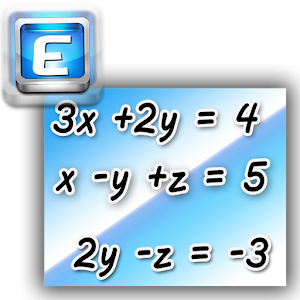## Linear Equation Solver helps you practice Grade 8 Math - Lumos Educational App Store1
Price -
\$

#### DESCRIPTION:

"Easy Equations : Linear Equation Solver" is a smart application which solves a system of linear equations and general day-to-day equations easier than ever. â Ability to solve system of linear equations up to 30 unknowns. â Fractional or decimal solution. â Shows how the problem is being solved (Row echelon form). â Ability to solve linear equations with complex numbers. â Ability to use your own variables in linear equations (written equations mode). You can use subscripted numbers also. ( Ex: a1, a2, a3 ..) â Ability to solving systems o

#### OVERVIEW:

Linear Equation Solver is a free educational mobile app By K. Dev.It helps students in grades 8 practice the following standards .

This page not only allows students and teachers download Linear Equation Solver but also find engaging Sample Questions, Videos, Pins, Worksheets, Books related to the following topics.

8

Developer: K. Dev

Software Version: 2.4.9

Category: Education

### RELATED APPS:EdSearch WebSearch